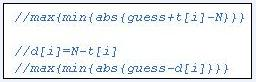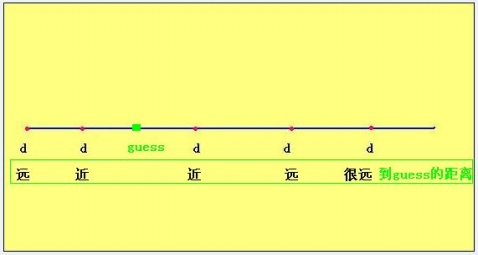# USACO[Elite 2010 March Competition/silver]

USACO终于升到金组了。本来该满分的，但是。。。CODE:

/*

PROG: rocks

LANG: C++

ID: boleyn.2

*/

/*

TITLE: The Rock Game [Tom Conerly, 2010]

CONTENT:

Before the cows head home for rest and recreation, Farmer John wants

them to get some intellectual stimulation by playing a game.

The game board comprises N (1 <= N <= 15) identical holes in the

ground, all of which are initially empty. A cow moves by either

covering exactly one hole with a rock, or uncovering exactly one

previously covered hole. The game state is defined by which holes

are covered with rocks and which aren’t. The goal of the game is

for the cows to reach every possible game state exactly once and

The cows have been having a tough time winning the game.  Below is

an example of one of their games:

Holes

time   1  2  3

—————–

0      O  O  O  Initially all of the holes are empty

1      O  O  X  The cow covers hole 3

2      X  O  X  The cow covers hole 1

3      X  O  O  The cow uncovers hole 3

4      X  X  O  The cow covers hole 2

5      O  X  O  The cow uncovers hole 1

6      O  X  X  The cow covers hole 3

7      X  X  X  The cow covers hole 1

Now the cows are stuck! They must uncover one hole and no matter

which one they uncover they will reach a state they have already

reached. For example if they remove the rock from the second hole

they will reach the state (X O X) which they already visited at

time 2.

Below is an example of a valid solution for N=3 holes:

Holes

time   1  2  3

—————–

0      O  O  O  Initial state: all of the holes are empty

1      O  X  O  The cow covers hole 2

2      O  X  X  The cow covers hole 3

3      O  O  X  The cow uncovers hole 2

4      X  O  X  The cow covers hole 1

5      X  X  X  The cow covers hole 2

6      X  X  O  The cow uncovers hole 3

7      X  O  O  The cow uncovers hole 2

8      O  O  O  The cow uncovers the 1st hole

which returns the game board to the start having, visited each state once.

The cows are tired of the game and want your help. Given N, create

a valid sequence of states that solves the game. If there are

multiple solutions return any one.

PROBLEM NAME: rocks

INPUT FORMAT:

* Line 1: A single integer: N

SAMPLE INPUT (file rocks.in):

3

OUTPUT FORMAT:

* Lines 1..2^N+1: A string of length N containing only ‘O’ and ‘X’

(where O denotes a uncovered hole and X denotes a covered

hole). The jth character in this line represents whether the

jth hole is covered or uncovered in this state. The first and

last lines must be all uncovered (all O).

SAMPLE OUTPUT (file rocks.out):

OOO

OXO

OXX

OOX

XOX

XXX

XXO

XOO

OOO

*/

#include <fstream>

using std::ifstream;

using std::ofstream;

using std::endl;

#include <cstring>

using std::memset;

class Application

{

ifstream cin;

ofstream cout;

static const int MAXN=15;

int N;

bool print(int state)

{

for (int i=0;i<N;i++)

if ((1<<i)&state) cout<<‘X’;

else cout<<‘O’;

cout<<endl;

return true;

}

void dfs(int step,int state)

{

static bool visited[1<<MAXN];

static bool finished;

if (finished) return;

if (step==(1<<N)&&(!state))

{

finished=true;

print(state);

return;

}

if (!visited[state])

{

visited[state]=true;

for (int i=0;i<N;i++)

if (!finished) dfs(step+1,state xor (1<<i));

visited[state]=false;

}

if (finished) print(state);

}

public:

Application(char* input,char* output)

:cin(input),cout(output)

{

cin>>N;

}

~Application()

{

cin.close();

cout.close();

}

int run()

{

dfs(0,0);

return 0;

}

};

int main()

{

Application app(“rocks.in”,“rocks.out”);

return app.run();

}

/*

PROG: teststr

LANG: C++

ID: boleyn.2

*/

/*

TITLE: Test Taking [Tom Conerly, 2010]

CONTENT:

Farmer John has to take his annual farming license test. The test

comprises N (1 <= N <= 1,000,000) true/false questions. After FJ’s

dismal performance on last year’s test Bessie wishes to help him.

Bessie has inside information that the number of questions whose

answer is true will be one of t_1, t_2, t_3,…, or t_K (0 <= t_i

<= N; 0 <= K <= 10,000) — even though Bessie has no information

about any answer to any specific question. Bessie wants to know the

best score that FJ is guaranteed achieve by exploiting this information

carefully, even if he doesn’t know the actual answers to any test

questions.

To demonstrate Bessie’s idea, consider a license test with N=6

questions where Bessie knows that the number of questions with the

answer ‘true’ is either 0 or 3. FJ can always get at least 3 answers

correct using logic like this: If FJ answers ‘false’ on every

question and there are 0 questions with the answer ‘true’ then he

will get all 6 correct. If there are 3 questions with the answer

‘true’ then he will get 3 answers correct. So as long as he marks

every answer as ‘false’ he is guaranteed to get at least 3 correct

without knowing any answer to the test questions.

On the other hand, consider what happens if FJ chooses an inferior

strategy: he guesses that some 3 answers are ‘true’ and the other

3 are ‘false’. If it turns out that NO answers are ‘true’ then FJ

will get 3 answers correct, the ones he guessed were false. If 3

answers are ‘true’ then FJ could get as few as 0 answers correct.

If he answered incorrectly on all 3 of that answers for which he

guessed ‘true’, and the other 3 (for which he guessed ‘false’) are

true, then he gets 0 correct answers. Even though FJ could get 3

correct in this case by guessing ‘false’ every time, he can not

always guarantee even 1 correct by guessing some 3 answers as ‘true’,

so this strategy can not guarantee getting any answer correct. FJ

should use the previous paragraph’s strategy.

Given Bessie’s inside information, determine the number of answers

FJ can always get correct using this information assuming he uses

it optimally.

PROBLEM NAME: teststr

INPUT FORMAT:

* Line 1: Two space-separated integers: N and K

* Lines 2..K+1: Line i+1 contains a single integer: t_i

SAMPLE INPUT (file teststr.in):

6 2

0

3

OUTPUT FORMAT:

* Line 1: A single integer, the best score FJ is guaranteed to achieve

SAMPLE OUTPUT (file teststr.out):

3

*/

#include <fstream>

using std::ifstream;

using std::ofstream;

using std::endl;

#include <cstring>

using std::memset;

#include <algorithm>

using std::sort;

class Application

{

ifstream cin;

ofstream cout;

static const int MAXK=10000;

int N,K;

int t[MAXK+2];

inline int abs(int n)

{

return n>-n?n:-n;

}

public:

Application(char* input,char* output)

:cin(input),cout(output)

{

cin>>N>>K;

for (int i=1;i<=K;i++) cin>>t[i];

}

~Application()

{

cin.close();

cout.close();

}

int run()

{

//max{min{abs{guess+t[i]-N}}}

//d[i]=N-t[i]

//max{min{abs{guess-d[i]}}}

int d[MAXK+2];

for (int i=1;i<=K;i++)

d[i]=N-t[i];

sort(d+1,d+K+1);

for (int i=1;i<=K;i++)

/*

for (int guess=0;guess<=N;guess++)

{

int min=N;

for (int i=1;i<=K;i++)

{

//cout<<guess<<“,”<<i<<“=abs(“<<guess<<“+”<<t[i]<<“-“<<N<<“) “<<abs(guess+t[i]-N)<<endl;

if (abs(guess+t[i]-N)<min) min=abs(guess+t[i]-N);

}

cout<<guess<<“min=”<<min<<endl;

}

*/

return 0;

}

};

int main()

{

Application app(“teststr.in”,“teststr.out”);

return app.run();

}

/*

PROG: sboost

LANG: C++

ID: boleyn.2

*/

/*

PROGRAM: \$PROGRAM

AUTHOR: Su Jiao

DATE: 2010-3-13

DESCRIPTION:

Need For Speed [Jeffrey Wang, 2007]

Bessie is preparing her race car for the upcoming Grand Prix, but

she wants to buy some extra parts to improve the car’s performance.

Her race car currently has mass M (1 <= M <= 1,000) and is able

to push itself forward with a force of F (1 <= F <= 1,000,000).

The Race Car Performance Store has N (1 <= N <= 10,000) parts

conveniently numbered 1..N. Bessie can buy as many or as few parts

as she wishes though the store stocks no more than one instance

of each part.

Part P_i adds force F_i (1 <= F_i <= 1,000,000) and has mass

M_i (1 <= M_i <= 1,000). Newton’s Second Law decrees that F =

MA, where F is force, M is mass, and A is acceleration. If Bessie

wants to maximize the total acceleration of her race car (and

minimize the mass otherwise), which extra parts should she choose?

Consider a race car with initial F=1500 and M=100. Four parts are

available for enhancement:

i  F_i  M_i

1  250   25

2  150    9

3  120    5

4  200    8

Adding just part 2, e.g., would result in an acceleration of

(1500+150)/(100+9) = 1650/109 = 15.13761.

Below is a chart of showing the acceleration for all possible

Parts   Aggregate   Aggregate

1234        F           M       F/M

0000      1500         100    15.0000

0001      1700         108    15.7407

0010      1620         105    15.4286

0011      1820         113    16.1062

0100      1650         109    15.1376

0101      1850         117    15.8120

0110      1770         114    15.5263

0111      1970         122    16.1475 <– highest F/M

1000      1750         125    14.0000

1001      1950         133    14.6617

1010      1870         130    14.3846

1011      2070         138    15.0000

1100      1900         134    14.1791

1101      2100         142    14.7887

1110      2020         139    14.5324

1111      2220         147    15.1020

Thus, the best additional part combination is parts 2, 3, and 4.

PROBLEM NAME: sboost

INPUT FORMAT:

* Line 1: Three space-separated integers: F, M, and N

* Lines 2..N+1: Line i+1 contains two space separated integers: F_i

and M_i

SAMPLE INPUT (file sboost.in):

1500 100 4

250 25

150 9

120 5

200 8

OUTPUT FORMAT:

* Lines 1..P: The index values of P extra parts, one per line, that

Bessie should add to her racecar. If she should not add any,

output ‘NONE’ (without quotes). The output should be given in

increasing order, so if the optimal set of parts is {2,4,6,7},

then the output should be in the order 2,4,6,7 and not, for

example, 4,2,7,6. Solutions will be unique.

SAMPLE OUTPUT (file sboost.out):

2

3

4

*/

#include <iostream>

using std::cin;

using std::cout;

using std::endl;

#include <fstream>

using std::ifstream;

using std::ofstream;

using std::endl;

#include <sstream>

using std::stringstream;

#include <vector>

using std::vector;

#include <string>

using std::string;

#include <stack>

using std::stack;

#include <queue>

using std::queue;

#include <map>

using std::map;

using std::pair;

using std::make_pair;

#include <algorithm>

using std::sort;

#include <cassert>

//using std::assert;

struct Compare

{

bool operator()(const pair<int,pair<int,int> >& a,

const pair<int,pair<int,int> >& b)

{

return (long long int)(a.second.first)*(long long int)(b.second.second)

>

(long long int)(b.second.first)*(long long int)(a.second.second);

}

}compare;

class Application

{

ifstream cin;

ofstream cout;

int N;

pair<int,pair<int,int> > FM;

vector<pair<int,pair<int,int> > > fm;

public:

Application(char* input,char* output)

:cin(input),cout(output)

{

cin>>FM.second.first>>FM.second.second>>N;

fm.resize(N);

for (int i=0;i<N;i++)

{

cin>>fm[i].second.first>>fm[i].second.second;

fm[i].first=i+1;

}

}

int run()

{

sort(fm.begin(),fm.end(),compare);

int i=0;

while (compare(fm[i],FM)&&(i<fm.size()))

{

FM.second.first+=fm[i].second.first;

FM.second.second+=fm[i].second.second;

i++;

}

fm.resize(i);

if (i)

{

sort(fm.begin(),fm.end());

for (int i=0;i<fm.size();i++)

cout<<fm[i].first<<endl;

}

else cout<<“NONE”<<endl;

return 0;

}

};

int main()

{

Application app(“sboost.in”,“sboost.out”);

return app.run();

}